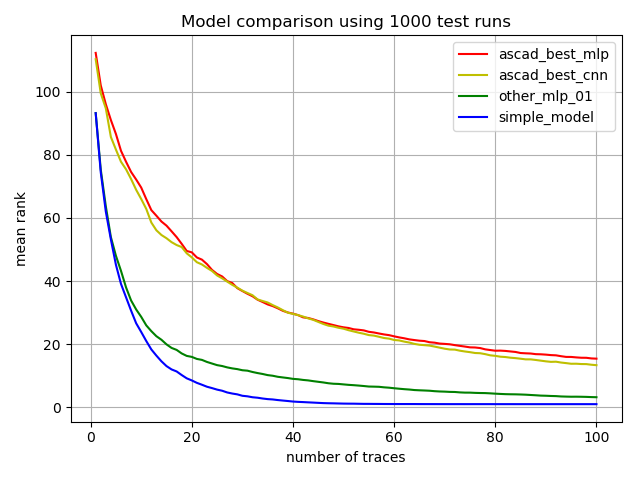# Simpler Models that fit ASCAD Data

Researchers at ANSSI and CEA-Leti have published an interesting paper on Deep Learning in Side Channel Analysis, along with measurement data and trained models, called the ASCAD database. Scripts and instructions how to obtain the data are found in a repository on GitHub.

Two simpler models that fit the ASCAD data quite well are provided below, with some scripts that allow comparison with the original ASCAD trained models.

To check out the simpler models, you can proceed as follows:
1. Download the ASCAD database as described in the README.md file in the ASCAD GitHub repository.
2. Install Python3 and packages required to work with the ASCAD models, in particular: numpy, matplotlib, h5py, keras, tensorflow, etc.
3. Download the following tarball from this website, containing the simpler models: simpler_models_20180527.tgz
4. Go to the ASCAD directory that contains the subfolder ASCAD_data with the ASCAD database and trained models, and unpack the contents of simple_model.tgz into that directory (i.e. above ASCAD_data). You will obtain the following new files:

 Filename Contents run_models.py Script that runs tests with two ASCAD models and the simpler models model_comparison.png Graphics output created with: python3 run_models.py 1000 100 AES_Sbox.py AES S-Box table used by run_models.py ascad_best_mlp.py Wrapper for ASCAD "best MLP" model ascad_best_cnn.py Wrapper for ASCAD "best CNN" model simple_model.py The simple model presented here simple_model.bin Numpy arrays used in the simple model mk_simple_model.py The script that was used to create simple_model.bin other_mlp_01.py Wrapper for another MLP model other_mlp_01.h5 Saved parameters for that other MLP train_other_mlp_01.py The script that was used to create other_mlp_01.h5 (will overwrite when re-run)

5. Run the test script with a command like:  python3 run_models.py
The script run_models.py performs, by default, 100 test runs with a test batch size of 70 on each model. These numbers can be modified by command-line arguments. In each run, a batch of randomly selected test traces is used, and the current model is used to predict probabilities for S-Box outputs for all traces in the batch. The rank of the correct subkey is then tracked as the evidence from each trace is cumulatively added in along the batch. Finally, a plot is produced that shows the mean rank numbers obtained for each number of traces, averaged over all test runs (batches).

If you are patient enough to run a command like  python3 run_models.py 1000 100,  you will be presented with a plot like the following:The first simpler model is implemented in simple_model.py as follows:
import numpy as np

f = open('simple_model.bin', 'rb')
pr = np.fromfile(f, dtype=np.float32, count=10*700).reshape(700,10)
mR = np.fromfile(f, dtype=np.float32, count=10*256).reshape(256,10)
mX = np.fromfile(f, dtype=np.float32, count=10*256).reshape(256,10)
f.close()

S = np.tile(np.arange(0x100, dtype = np.uint8).reshape(1,256), (256,1))
R = np.tile(np.arange(0x100, dtype = np.uint8).reshape(256,1), (1,256))
X = S ^ R

m = mR[R,:] + mX[X,:]

def predict(batch):
upr = batch.dot(pr).reshape(-1,1,1,10)
return np.exp(-.5 * ((upr - m) ** 2).sum(3)).sum(1)

Assembling a pile of numbers as in simple_model.bin is left as an exercise to the reader (it is less than 48 KByte), or you may use the script mk_simple_model.py supplied in the tarball to learn how it was done.

The second simpler model is another MLP model which uses 5 hidden layers with 50 units each. It was trained by running train_other_mlp_01.py, iterating over 25 epochs. In terms of Keras statements its layout is defined as follows:
m = Sequential([
BatchNormalization(input_shape=(700,), trainable = False),
Dense(50, activation='relu'),
Dense(50, activation='relu'),
Dense(50, activation='relu'),
Dense(50, activation='relu'),
Dense(50, activation='relu'),
Dense(256, activation='softmax')
])

A non-trainable batch normalization layer is used on the input, initialized with means and standard deviations of the training set. Without normalization, about half the neurons in the first layer would be "dead", i.e. they would have zero activation for all traces in the dataset. The normalization also allows the optimizer to work more efficiently. This may be why the model gets away with only 50 units per layer and with only 25 epochs of training, while still showing reasonable performance.